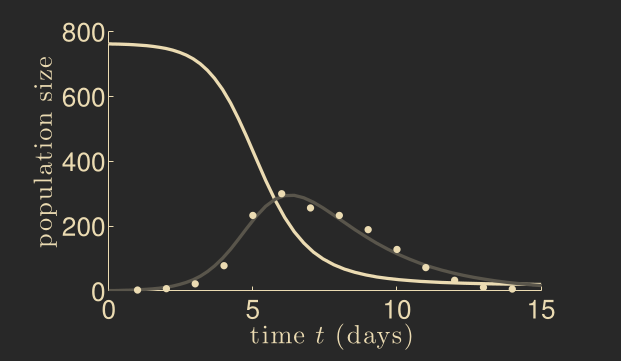# Struggling with Passing Multiple Initial Conditions to ODE

Hi folks,Been doing a lot of work with the great SciML ecosystem and building more familiarity with its offerings.

I am struggling with an ODE that I am trying to model. Here is the equation:

\frac{dI}{dt} = a \cdot \frac{B}{k + B} \cdot S - r \cdot I

Which I wrote in the function:

I(a, k, r, S, B, I) = (a * S) * (B / (k + B)) - r * I


As a, k, and r are constants, I replaced them in my formulation as follows:

I(S, B, I) = (1 * S) * (B / (10e6 + B)) - 0.2 * I


For this ODE, I had the initial values for S, B, and I:

initial_values =
[
H - 1, # S(0)
0, # B(0)
1, # I(0)
]


Where H is a population count comes from me looping over a set number of population counts. I read up on how to pass multiple parameters to my ODE using DifferentialEquations.jl and came up with a final function formulation that looked like this:

I(I, p, t) = ((a_1 * p) * (p / (k_1 + p))) - (r_1 * p)


My full code is as follows:

# Setting up labeling of the figure
fig = Figure(fontsize = 24);
ax = Axis(fig[1, 1],
title = L"Plot of $I$",
xlabel = L"t",
ylabel = L"Population Size"
);

# Time interval from $0$ to $20$ days
tspan = (0, 20)

# Total human populations
populations = [5000, 6000, 7000, 8000, 9000, 10000]

# ODE I am evaluating
I(I, p, t) = ((a_1 * p) * (p / (k_1 + p))) - (r_1 * p)

# Looping through different population sizes
for H in populations

initial_values =
[
H - 1, # S(0)
0, # B(0)
1, # I(0)
]

# Set-up ODE
prob = ODEProblem(I, 1, tspan, initial_values)

# Use Tsit5 solver for non-stiff ODEs
sol = solve(prob, Tsit5(), reltol = 1e-8, abstol = 1e-8)

lines!(ax, sol.t, sol.u, label = L"H = \$H")
end

# Show plot
fig


However, the figure output (below) doesn’t seem to be matching what I expect (next figure).Even though the values aren’t exactly the same (they shouldn’t be since I am looking at different populations), they are all coming out to the same line and I don’t see any behavior variation that I would expect like in the second figure.

Could anyone point out to me what I may be doing wrong with my ODEProblem set-up? I was thinking I am somehow screwing up my implementation but unsure where.

Thanks!

~ tcpP.S. For full transparency, this is part of a homework assignment but I am allowed collaboration.

Be careful mixing your states and parameters. States evolve in time and parameters are static.

I think you want something like

function eom(I, params, t)
a, k, r, S, B = params
(a * S) * (B / (k + B)) - r * I #note r*I, where I is from the first argument of eom not params
end

initial_condition = 1.0
params = ... # set to your static values for as (a, k, r, S, B)
prob = ODEProblem(eom, initial_condition, tspan, params)



It seems like what you call “initial_values” are actually static parameters. In your function I(I, p, t) = ((a_1 * p) * (p / (k_1 + p))) - (r_1 * p) you are using p for I. But that is a state. The variables p do not change.

2 Likes

I think @DrPapa is spot on. I just wanted to add that it is easy to get confused when naming a function the same as one of its arguments:

I(I, p, t) = ((a_1 * p) * (p / (k_1 + p))) - (r_1 * p)


Since the function is actually supposed to computes \frac{\mathrm{d}I}{\mathrm{d}t}, perhaps dI might be better (I also included the previously mentioned fix turining p into I):

dI(I, p, t) = ((a_1 * p) * (p / (k_1 + p))) - (r_1 * I)


Also, your model looks somewhat similar to the SIR models from epidemiology. Perhaps this repository is a useful resource to get started using ODE solvers specifically for the SIR family of models: GitHub - epirecipes/sir-julia: Various implementations of the classical SIR model in Julia

E.g. this notebook.

EDIT: Just noticed that there is also a dedicated tutorial folder in the repository with more detailed explanations https://github.com/epirecipes/sir-julia/blob/master/tutorials/ode/ode.jmd

1 Like